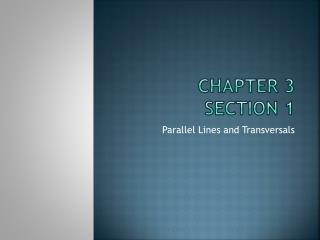# Chapter 3 Section 1 - PowerPoint PPT PresentationDownload PresentationChapter 3 Section 1

Chapter 3 Section 1Download Presentation## Chapter 3 Section 1

- - - - - - - - - - - - - - - - - - - - - - - - - - - E N D - - - - - - - - - - - - - - - - - - - - - - - - - - -
##### Presentation Transcript

1. Chapter 3Section 1 Parallel Lines and Transversals

2. Warm-Up L M N Q P 1) Are points L, M, and Q collinear? 2) Find the measure of MN if LM = 5x – 4, MN = 6x + 1, and LN = 30? 3) Name an acute angle, an obtuse angle, and a right angle in the figure. 4) Are <LMP and <NMQ complementary?

3. Warm-Up L M N Q P 1) Are points L, M, and Q collinear? No because they are not on the same line.

4. Warm-Up L M N Q P 2) Find the measure of MN if LM = 5x – 4, MN = 6x + 1, and LN = 30? Use the segment addition postulate. LN = LM + MN 30 = 5x – 4 + 6x + 1 30 = 11x – 3 33 = 11x 3 = x Plug 3 in for x in the equation for MN. MN = 6x + 1 MN = 6(3) + 1 MN = 18 + 1 MN = 19

5. Warm-Up L M N Q P 3) Name an acute angle, an obtuse angle, and a right angle in the figure. Acute angle - <LPM or <QMN Obtuse angle- <PMN or <LMQ Right angle- <PMQ 4) Are <LMP and <NMQ complementary? Yes because they form a straight line with <PMQ. So <LMP + <NMQ + <PMQ = 180 <LMP + <NMQ + 90= 180 <LMP + <NMQ = 90 (Definition of complementary)

6. Vocabulary Parallel lines: Two lines that never meet. (Lines l and m are parallel) Skew lines: Two lines are skew if they do not intersect and are not in the same plane. Transversal: A line that intersects two or more lines in a plane at different points. (Line t is the transversal) Exterior Angles- In the figure, transversal t intersects lines l and m. The exterior angles are <3, <4, <5, and <6. Interior Angles- In the figure, transversal t intersects lines l and m. The interior angles are <1, <2, <7, and <8. t 5 6 l 7 8 1 2 m 3 4

7. Vocabulary Cont. Consecutive Interior Angles- In the figure, transversal t intersects lines l and m. <7 and <1, and <8 and <2 are consecutive interior angles. Alternate Interior Angles- In the figure, transversal t intersects lines l and m. <7 and <2, and <8 and <1 are alternate interior angles. Alternate Exterior Angles- In the figure, transversal t intersects lines l and m. <5 and <4, and <6 and <3 are alternate exterior angles. Corresponding Angles- In the figure, transversal t intersects lines l and m. <5 and <1, <7 and <3, <6 and <2, and <8 and <4 are corresponding angles. t 5 6 l 7 8 1 2 m 3 4

8. Example 1: Refer to the figure at the right. • a) Name all planes parallel to the plane ADH. • Plane BCG • b) Name all the segments that intersect AT. • Line segments AB, AC, and AD • c) Name all the segments that are parallel to AT. • Line segments DH, BK, and CG • d) Name all segments that are skew to CG. • Line segments TK, TH, AD, and AB B C A D G K T H

9. Example 2: Refer to the figure at the right. • a) Name all planes that are parallel to plane ABC. • Plane TKG • b) Name all segments that intersect AB. • Line segments BC, AC, AD, AT, and BK • c) Name all the segments that are parallel to KG. • Line segments BC, AD, and TH • d) Name all segments that are skew to TK. • Line segments CG, DH, AD, AC, and BC B C A D G K T H

10. Example 3: Identify each pair of angles as alternate interior, alternate exterior, corresponding, or consecutive interior angles. • a) <1 and <8 • Alternate Exterior Angles • b) <7 and <10 • Alternate Interior Angles • c) <8 and <12 • Corresponding Angles • d) <1 and <5 • Corresponding Angels • e) <4 and <6 • Consecutive Interior Angles • f) <8 and <9 • Alternate Interior Angles l 1 2 3 4 6 5 8 7 10 9 m 11 12 t

11. Example 4: Identify each pair of angles as alternate interior, alternate exterior, corresponding, or consecutive interior angles. • a) <6 and <10 • Consecutive Interior Angles • b) <9 and <11 • Alternate Interior Angles • c) <1 and <5 • Corresponding Angles • d) <3 and <8 • Alternate Exterior Angels • e) <7 and <12 • Alternate Interior Angles • f) <4 and <8 • Corresponding Angles m l 1 8 10 2 5 t 11 4 12 6 7 3 9

12. Example 5: Refer to the figure showing three lines and the angles formed by these lines. • A) Identify the transversal to lines l and m. • Line t • B)Identify the special name given to each pair of angles • <7 and <12 • Corresponding Angles • <8 and <10 • Alternate Interior Angles • <2 and <12 • Alternate Exterior Angles l m 1 9 6 11 2 8 4 5 t 3 7 10 12